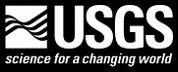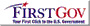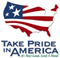PUBLICATIONS—Open File Report

# A Semi-Implicit, Three-Dimensional Model for Estuarine Circulation

By Peter E. Smith

U.S. GEOLOGICAL SURVEY

Open-File Report 2006-1004

Sacramento, California 2006

Prepared in cooperation with the Interagency Ecological Program for the San Francisco Bay-Delta Estuary

Complete accessible text of report (6.3 MB PDF)

## Abstract

A semi-implicit, finite-difference method for the numerical solution of the three-dimensional equations for circulation in estuaries is presented and tested. The method uses a three-time-level, leapfrog-trapezoidal scheme that is essentially second-order accurate in the spatial and temporal numerical approximations. The three-time-level scheme is shown to be preferred over a two-time-level scheme, especially for problems with strong nonlinearities. The stability of the semi-implicit scheme is free from any time-step limitation related to the terms describing vertical diffusion and the propagation of the surface gravity waves. The scheme does not rely on any form of vertical/horizontal mode-splitting to treat the vertical diffusion implicitly. At each time step, the numerical method uses a double-sweep method to transform a large number of small tridiagonal equation systems and then uses the preconditioned conjugate-gradient method to solve a single, large, five-diagonal equation system for the water surface elevation. The governing equations for the multi-level scheme are prepared in a conservative form by integrating them over the height of each horizontal layer. The layer-integrated volumetric transports replace velocities as the dependent variables so that the depth-integrated continuity equation that is used in the solution for the water surface elevation is linear. Volumetric transports are computed explicitly from the momentum equations. The resulting method is mass conservative, efficient, and numerically accurate.

## CONTENTS

Abstract

1. Introduction

2. Governing Equations and Boundary Conditions

3. Layer Averaging the Governing Equations

4. Finite-Difference Formulation

5. Numerical Experiments

6. Summary and Conclusions

7. References

Appendix A. Coriolis Acceleratin

Appendix B. Equation of State

Appendix C. The σ Transformation

Appendix D. Expansion of Explicit Finite-Difference Terms

Appendix E. Computation of the Vertical Eddy Coefficients

 AccessibilityFOIAPrivacyPolicies and Notices U.S. Department of the Interior, U.S. Geological Survey Persistent URL: http://pubs.water.usgs.gov/ofr20061004 Page Contact Information: Publications Team Last modified: Saturday, 12-Jan-2013 22:01:19 EST